# Progressive & Stationary Waves

## Progressive Waves

A progressive wave oscillates the particles of the medium it is travelling through. A transverse waves has oscillations that are perpendicular to the direction of energy transfer whereas longitudinal oscillate parallel.

Key Terms

Amplitude: The maximum displacement from the equilibrium position

Frequency: How many waves are produced per second

Speed: The distance a wave covers over a given time frame

Phase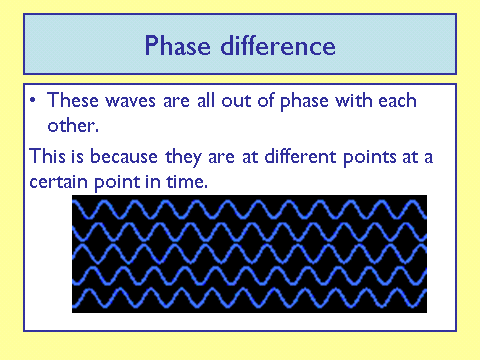Radians

As mentioned above, phase can be measured by time, degrees or radians.

One radian is the angle that subtends a distance on the circumference on a circle that is equal to the radius of that circle.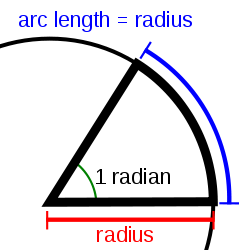Radians can be given in terms of 𝝅.

Common conversions are:

90o = 𝝅/2

180o = 𝝅

360o = 2𝝅

You can easily work out any conversion knowing the ratio that:

1o = 𝝅/180

Convert 2𝝅/5 in degrees
72

## Longitudinal & Transverse Waves

Transverse Waves:

A transverse wave is a wave that oscillates perpendicular to its propagation

OR

A wave that vibrates at right angles to the direction of energy transfer.

A transverse wave looks like this:Don’t forget to always include the labels!

Examples include ripples on a water surface and an electromagnetic wave (light)

Longitudinal Waves:

A longitudinal wave is a wave that oscillates parallel to its propagation

OR

A wave that vibrates parallel to the direction of energy transfer.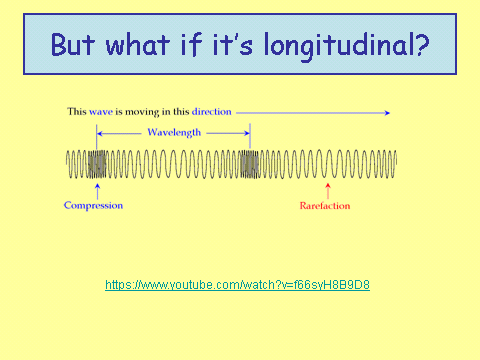Don’t forget to always include the labels!

An example of this wave is a sound wave

Displacement of Particles

Exam questions often require you to apply the direction of displacement of particles/fields relative to the direction of propagation. These occurs most often for transverse waves.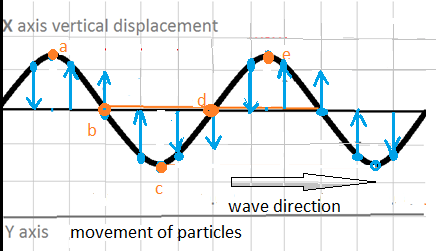In the diagram above looking at point c, we notice that as the wave progresses particles at point c will rise through the equilibrium position up to the crest of the wave. Take a moment to see how all the other points move for half a wave cycle.

Polarisation

Polarisation is a phenomenon that can only occur in transverse waves.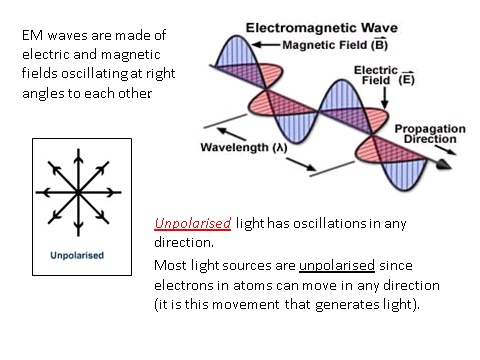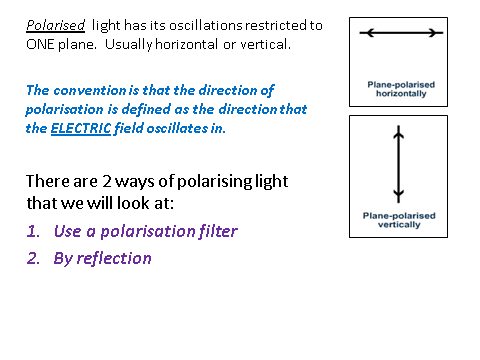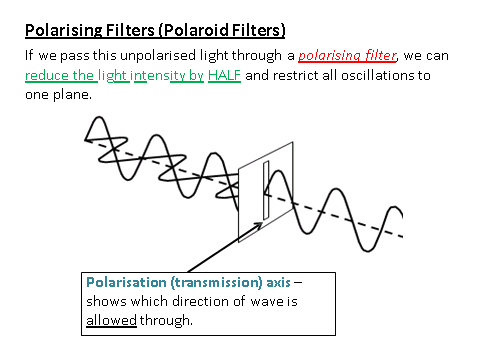Application of Polarisation

Polarisation has a number of different useful application:.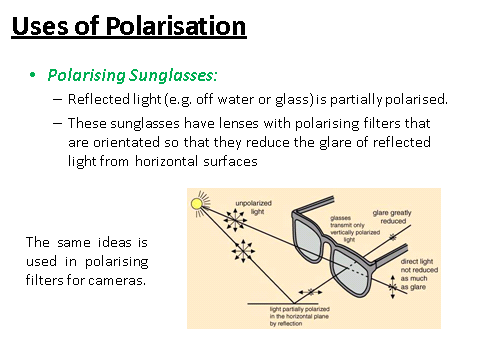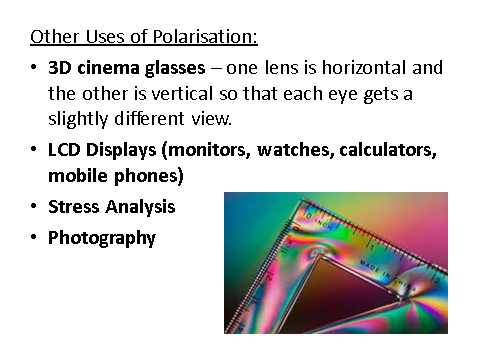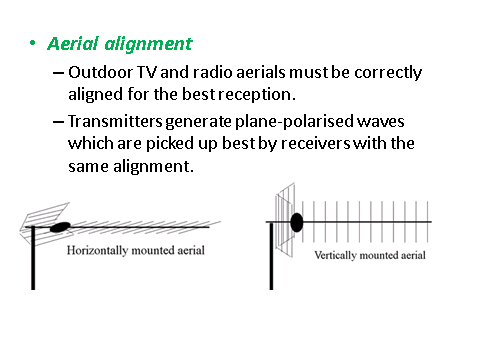True or False - Sound waves can be polarised.
False
Using the diagram under Displacement of Particles, describe the motion of point E for a quarter wave cycle.
Your answer should include: Moves Down / Equilibrium Position
Explanation: Moves down to the equilibrium position.

## Superposition

Stationary Waves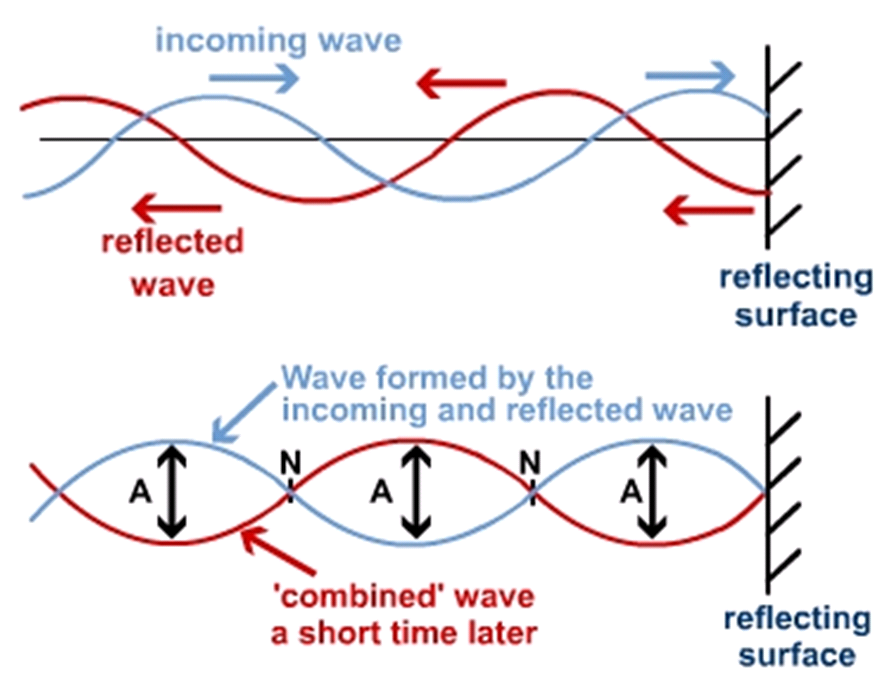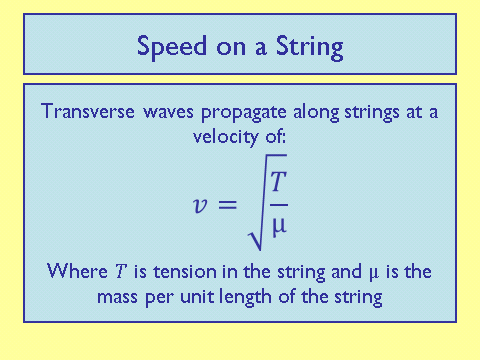Stationary waves can occur in all waves, transverse and longitudinal.

First Harmonic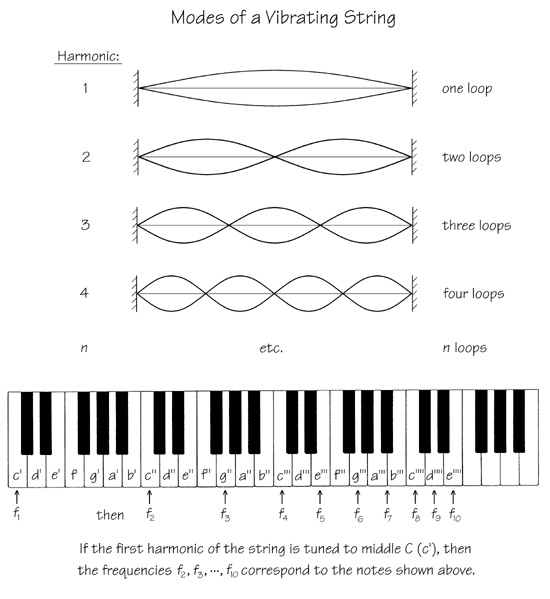The first harmonic is shown in the diagram above. The wavelength is twice the distance between the two nodes

The formula for the frequency is:

__Ff __= frequency (Hz)

Ll = length of string (m)

__T __= Tension (N)

𝝁 = mass per unit length (kgm-1)

Harmonics

It is possible to get more harmonics as shown in the diagram below. As you move from left two right you start with the first harmonic, then the second (a full wavelength), third etc.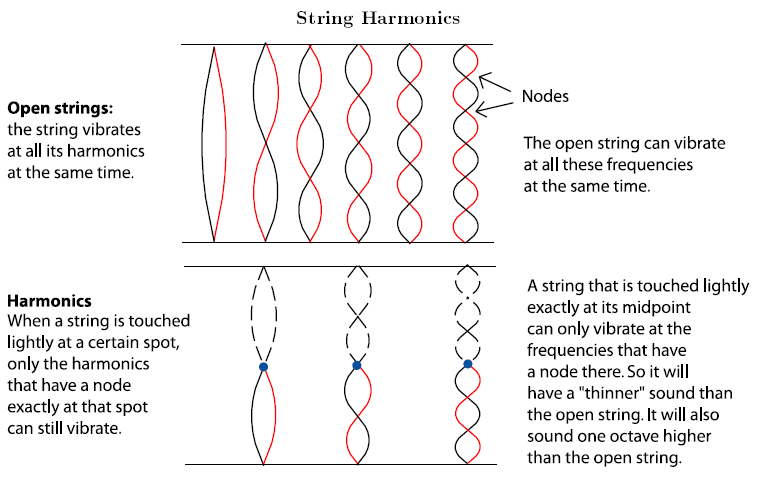Questions are often around describing how a stationary wave is formed, using the equation for the first frequency and applying a stationary wave to difference scenarios. It is vital to use scientific terminology (such as nodes and antinodes) in your answers.